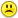+0

# Help

0
361
4

The graph of the function $$y=f(x)$$ is shown below. For all $$x > 4$$, it is true that $$f(x) > 0.4$$. If $$f(x) = \frac{x^2}{Ax^2 + Bx + C}$$, where $$A,B$$ and $$C$$ are integers, then find $$A+B+C$$.Jul 14, 2019

#1
+3

goodbye web2calc

.
Jul 14, 2019
edited by Rom  Jul 14, 2019
#3
+4

Goddamn you ROM! If you leave, the forum’s mean IQ will drop by 30 bloody points.

Just take a bleedin’ break, and visit when you have a mind too.

Take your boat to Gilligan’s Island; after a few weeks there this place won’t seem so annoying.

I’m NOT the only one who feels this way!

GA

For the past 5 hours, I have pondered why Rom posted his “goodbye” by editing and replacing a fine teaching post.

I didn’t save a copy, but the post described how to correlate the graphical locations of the asymptotes to an equation.  This was an excellent, wonderful post –not a bloody thing a matter with it. Now it’s gone. Replaced with a “goodbye...”

This post was chosen deliberately (it wasn’t his latest post).  I know why too....

GingerAle  Jul 15, 2019
edited by GingerAle  Jul 15, 2019
#4
+5

I agree besides Melody and Chris aka CPhill , and EP, their is no one to answer all the hard questions

DON'T LEAVE ROM!

travisio  Jul 15, 2019
edited by travisio  Jul 15, 2019
#2
+2

Because it appears that  (0,0)  is on the graph, the numerator has the form Dx^2

Because we have vertical asymptotes  at x  = -2  and x = 3.....the denominator has the form

A (x + 2) (x - 3)

And the line y = 0.4 = 2/5  is an asymptote  for x > 4......so  we might guess that the ratio of D/ A  = 2/5

So....it appears that we have

2x^2                    2x^2                          2x^2

f(x)  =    ______________ =     ______________ =    _________________

5 ( x + 2) (x - 3)           5 (x^2 - x - 6)             5x^2 - 5x - 30

So A + B + C  =   5 - 5 - 30   =    -30

Note that when  x < -6, the graph  will cross the asymptote of y = 2/5

Here's a graph :  https://www.desmos.com/calculator/7i9wnrvuucJul 14, 2019
edited by CPhill  Jul 14, 2019